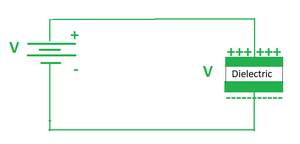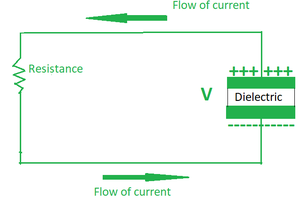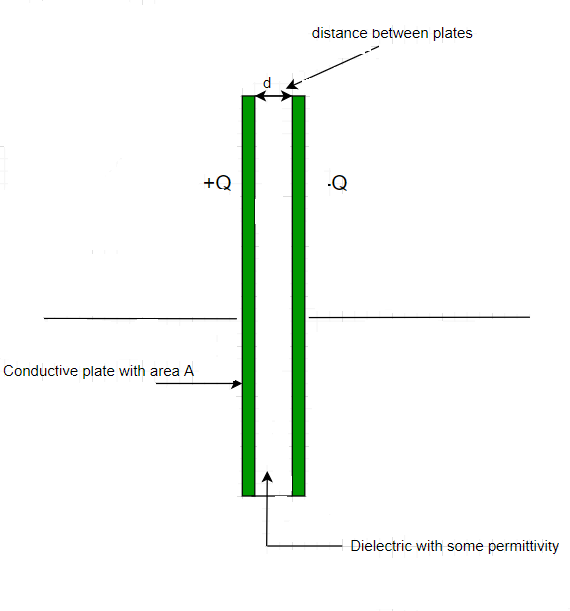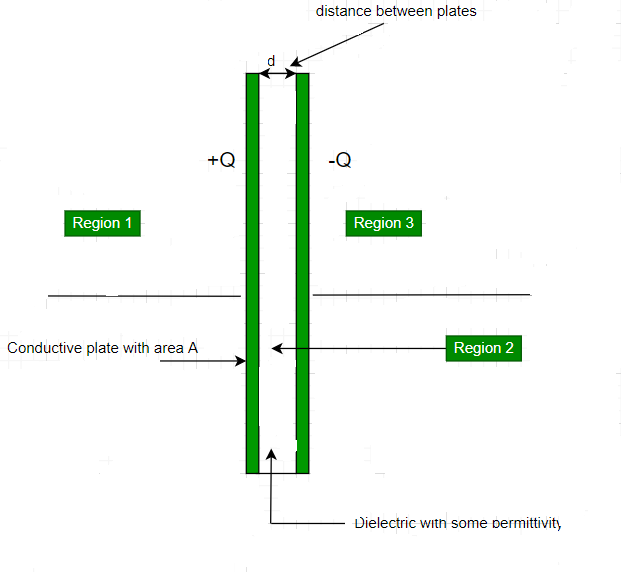GFG App
Open AppBrowser
Continue

# What is a Parallel Plate Capacitor?

Capacitance can be defined in Layman’s terms as a physical quantity that indicates the ability of a component or circuit to collect and store energy in the form of an electrical charge. So we can say that a capacitor is an electrical component that draws energy from a battery and stores the energy. Then the terminals can be connected to a circuit having some load resistance. When this is done the capacitor quickly releases electricity in a tiny fraction of a second. A capacitor can be made in any way however there are always two requirements that need to be completed

• It should be made of two terminals which are of conducting nature
• There needs to be some gap between the two terminals and that gap should be filled with a dielectric (insulating).

Generally in a capacitor, the two terminals are made up of some conducting material like thin foil, aluminum, and the dielectric can be made of glass or mica, or even air. A capacitor has many characteristics that make it an important part of a circuit.

• Since the charge is stored directly into the plates the charging and the discharging process become simple and easy.
• It is used as a filter as it allows AC to flow but not DC
• The capacitor can withstand high potential difference in a circuit and not breakdown
• Capacitors are useful in filtering out any unwanted frequencies.

### Working of a Capacitor

Consider a setup of two terminals separated by some distance and there is some medium (insulating in nature) between them now as we pass current through them the current will not flow due to the fact that there is some insulating material separating these two terminals from each other. The setup continues getting charged for quite some time such that both the terminals gain opposite charges and then it reaches a state where it has reached a point of maximum charge. The setup is called a capacitor and this time period is called the charging time of the capacitor.Fig 1. A capacitor is connected to a battery.

Later we can remove the battery from the capacitor and then place a load resistor into that circuit this would lead to the capacitor acting as a battery in a closed circuit and the current would flow from the positively charged terminal of the capacitor to the negatively charged terminal of the capacitor. And with time the capacitor would lose all of its charges. The time period in which it loses all of its charges is called the discharging period of the capacitor.Fig 2. Discharging phase of a capacitor.

Thus we can say that a capacitor is a device that can store charge and in turn energy in itself.

### Capacitance of a conductor

Consider a capacitor whose positive and negative plates hold +q and -q charge respectively. A DC voltage source is applied across it which gives it a potential difference of V across the plates. Now as we know,

Q ∝  V

Putting a constant C in this relation.

Q = CV.

Note: The capacitance of every material is unique and its SI unit is Farad (F).

### Parallel Plate capacitor

Consider a given setup of two plates that are connected across a battery, the arrangement is such that both the plates have the same physical dimensions and they are both placed parallel to each other and separated by some distance. The separation between the two plates is filled with some dielectric. The two plates are also oppositely charged since the plate connected to the positive terminal acquires a positive charge and the plate connected to the negative terminal acquires a negative chargeFig 3. A simple parallel plate capacitor.

In our case the area of both the plates is A and the charge on them is +Q and -Q. Also, the distance between them is given by d which is negligibly small when compared to the area of the plates so we can say that d<<A.

Now since d<<A.

Therefore both the plates can be considered as infinite plane sheets.

The surface charge density of positively charged plate can be given by.

The surface charge density of negatively charged plate can be given by.

Let’s assume that the parallel plate capacitor divides the plates into three regions in such a way that,

• The region to the left of the positively charged plate is Region 1.
• The region in the middle of the two plates is region 2.
• And the region to the right of the negatively charged plate is region 3.Fig4. The plane gets divided into three regions.

Now let’s calculate the electric fields in each of the three regions separately.

• Region 1:

Using Gauss’s law the electric field generated by an infinite sheet is given by:-.

The electric field generated by both the plates in region 1 is the same in magnitude and opposite in direction.

E = E1 + E.

E = 0.

• Region 2:

In this case we calculate the electric field in between the two plates.

Here the magnitude and the direction of the electric field are the same. So the generated electric field is given by:-

E =.

• Region 3:

This region is to the right of the negatively charged plate.

The electric field in this case is given by

E =.

E = 0.

Now the expression for capacitance is given as:. ⇢ (1)

The value of potential difference V can be found using the formula,

V = E × d.. ⇢ (2)

So the capacitance of the parallel plate capacitor can be given by

From (1) and (2),.

### Sample Questions

Question 1: A parallel plate capacitor is placed in the air. Find its capacitance if the area of each plate is 100 cm2 and the separation between the plates is 1 cm.

Solution:

The capacitance of the parallel plate capacitor can be given as,.

Here A = 100 ×10-4 m2, d= 10-2 m.

C =C = 8.85 × 10-12 F.

Question 2: A capacitor of area 100 cm^2 generates a capacitance of 17.7 × 10-11 F . Find out the separation between the plates of this capacitor.

Solution:

C =Here A = 100 × 10-4 m , C =  17.7 × 10-11 F

17.7 × 10-11  =d =  5 × 10-4  m.

Question 3: The area of a parallel plate capacitor is doubled and separation between the plates is halved to find out the change in its capacitance.

Solution:

C1According to question,

d2 =A2 = 2 A1

C2C2 = 4C2 = 4 C1

Question 4: A parallel plate capacitor with a plate area of 100 cm2 and separation between the plates of 1 cm is placed in the air is given a voltage of 1000V Find its energy

Solution:

The capacitance of the parallel plate capacitor can be given as,

C =Here A = 100 × 10-4m2, d = 10-2

C =C = 8.85 × 10-12 F.

The energy of this capacitor can be calculated by using the expression

U = 1/2 CV2

U =U = 4.425 × 10-6 J

Question 5: The capacitance of a parallel plate capacitor is 4.425 × 10-12 F. The separation between the plates is 1cm. Find the area of each plate.

Solution:

The capacitance of the parallel plate capacitor can be given as

C =Here C = 4.425 × 10-12 F , d= 10-2 m

4.425 × 10-12 = 8.85 × 10-12 × A/10-2   .

A =A = 5 × 10-3 m2

Question 6: A parallel plate capacitor is placed in the air. Find its capacitance if the area of each plate is 100 cm2 and the separation between the plates is 1 cm. If a voltage of 100 V is supplied how much charge will the capacitor store?

Solution:

the capacitance of the parallel plate capacitor can be given as

C =Here A = 100 × 10-4 m2, d = 10-2m.

C =.

C= 8.85 × 10-12   F.

The charge on a Capacitor is given by

Q = CV.

Q = 8.85 × 10-12 × 100 C.

Q = 8.85 × 10-10

Question 7: The separation between the plates of a parallel plate capacitor is filled with a material of Relative permittivity five times that of air find out its capacitance if the separation between the plates is 1 cm and the area of each plate is 200cm2.

Solution:

the capacitance of the parallel plate capacitor can be given as

C =Here A = 200 × 10-4 m2, d = 10-2m .

ε = 5 × 8.85 × 10-12  .

C =.

C = 8.85 × 10-11F .

My Personal Notes arrow_drop_up